# Performance of Solar Pond Integrated with Thermoelectric Generator: A Theoretical Study

Performance of Solar Pond Integrated with Thermoelectric Generator: A Theoretical Study
Mechanical Engineering Department, College of Engineering, University of Kerbala, Karbala 56001, Iraq

Corresponding Author Email:
basim.s@s.uokerbala.edu.iq
Page:
509-514
|
DOI:
https://doi.org/10.18280/mmep.100217
7 August 2022
|
Revised:
12 January 2023
|
Accepted:
20 January 2023
|
Available online:
28 April 2023
| Citation

OPEN ACCESS

Abstract:

This article intends to theoretically propose and investigate the construction of a solar pond with a salinity gradient and the generation of electrical energy from it using a thermoelectric generator. It is possible to gradually add salt (magnesium sulfate salt) to the lower layer, increasing the quantity of thermal energy supplied to the pond. According to the theoretical findings, increased heat storage in the pond’s bottom layer is enhanced by the addition of salt, and reflecting mirrors help to raise the quantity of solar radiation that is directed at the pond’s surface, increasing the amount of thermal energy that is absorbed through the layers of the pond. Thermal energy losses are decreased by the plastic cover that has been applied to the pond’s surface. When salt, a cover, and mirrors were added to the conventional pond, the amount of useable energy increased from (2 kilowatts) to (20 kilowatts), and these modifications helped the pond’s thermal efficiency rise from (5 percent) to (28.4 percent). TEG Electric Efficiency was 12% at a temperature of 65℃ and a mass flow rate of 2 L/min.

Keywords:

solar pond, magnesium sulphate, reflectors, TEG

1. Introduction

The current work aims to provide a theoretical study to improve the performance of the solar pond with salt (magnesium sulfate) and the addition of reflective mirrors, this to conserve thermal energy in the solar pond's bottom layer. As well as verifying the possibility of benefiting from the thermal energy of the pond to produce electrical energy using a thermoelectric generator (TEG). This is done by feeding TEG from one side with hot water produced from the solar pond in theory, while cold water is pumped from the other side TEG to create a temperature difference on both ends TEG to produce an electrical potential difference to be used in certain applications. This work is theoretically carried out in the city of Karbala, Iraq.

2. Solar Pond Construction

The test rig for the current investigation is a solar pond with a salinity gradient. The solar pond’s construction was raised by the following measures: Reflective mirrors are added once the plastic covering applies to the pond’s sides. Finally, the inside and edges of the pond were covered with glass, mirrors, and black stones. In Figure 1, the schematic diagram is shown. The pyramidal design and salt gradient were used in the production of the solar pond. The pond is 6.25 square meters on top, 1.35 meters deep, and 0.64 square meters at its bottom. There is a 60-degree tilt in the pond’s walls.

## 1.png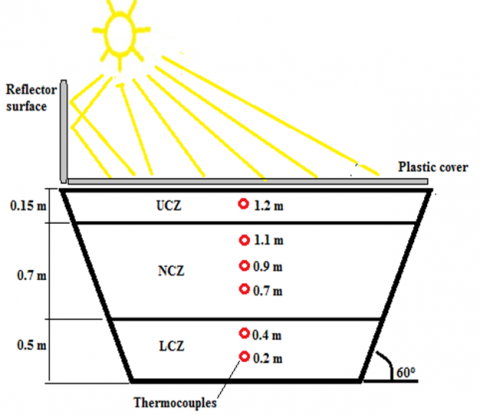Figure 1. A solar pond schematic with secondary, measurement, and modification devices

3. Mathematical Modelling

Heat Analysis of Lower Convective Zone:

$H_L=Q_{\text {cond. } 4}+\left(\frac{\partial T}{\partial t} * \rho_{L C Z} * C p_{L C Z} * X_{L C Z}\right)$     (1)

where, HL is the thermal energy entered into the lower convective zone Qcond.4 is the thermal energy exited from the lower zone $\frac{\partial T}{\partial t} * \rho_{L C Z} * C p_{L C Z} * X_{L C Z}$  is the thermal energy kept in the lowest zone of the solar pond.

$Q_{\text {cond.4 }}=-K_{L C Z} \frac{\partial T_n^t}{\partial x} \mid$     (2)

The finite difference of brine temperature of the pond lower zone (LCZ) can be determined by the next equation:

$T_L^{t+1}=T_L^t+\frac{\Delta t}{\rho_{L C Z} \, \, \, \, * \, C p_{L C Z} \, \, \, \, * \, X_{L C Z}} \, \, \, \left[\left(H_L\right)+\frac{K_{L C Z} \, \, \, \left(T_L^t+T_n^t\right)}{X_{L C Z} \, \, / 2}\right]$     (3)

The solar pond useful thermal energy (Qu) can be determined by the following equation :

$Q_u=M_s C p_s \Delta T$     (4)

where, Ms is the solar pond brine mass of, Caps is the pond brine heat capacity of and ΔT is the average temperature difference between the ambient and lower zone.

The solar pond thermal efficiency (Ƞ) can be calculated by division of the useful energy (Qu) on the quantity of incident sun light on the pond surface (I) as follows :

$\eta=Q_u / I$      (5)

The incident sun light on the pond surface (I) can be found by the following equation:

$I=I_O * A$     (6)

where, A is the solar pond surface area.

TEG electrical power generated is given as studies [19-23]:

$P_{T E G}=I * V$     (7)

$V_{T E G}=\alpha * \Delta T$     (8)

where, (αpαn)=α and (ThTc)=ΔT, Tc and Th denote the cold and hot junction temperature. αp and αn are the Seebeck coefficient of P and N type TEG leg respectively.

The following equation may represent short circuit and open circuit relationships in a TEG with N number of couples.

$V_{T E G}=2 * N * \alpha * \Delta T$      (9)

$I_{T E G}=\left(\frac{\alpha}{\rho}\right) *\left(\frac{A_{T E G}}{L}\right) * \Delta T$     (10)

Electrical resistivity of thermo element material, (ρ Ω cm). Area of thermo element, (ATEG mm2). Length of thermo element, L mm.

The thermoelectric power is related to dimensionless figure of merit given by:

$Z T=\alpha^2 * \sigma * T / K$      (11)

where, α is the TEG Seebeck coefficient, k and σ are the thermal conductivity and electrical and T is the absolute temperature.

For calculating the efficiency of TEG, the following equation is used.

$\eta_{T E G}=\left(T_h-T_C\right) / T_h * \frac{(1+Z T)^{0.5}-1}{(1+Z T)^{0.5}+\left(\frac{T_C}{T_h}\right)}$     (12)

4. Theoretical Results

In theory, the study is carried out using the MATLAB program to resolve the governing equations and constrained flowcharts in this program such as theories of solar radiation falling on the solar pond surface and the mathematical calculations to compute it. It also includes analyzes of heat transfer through the lower layer of the solar pond. In addition, the mathematical equations for calculating the heat loss and the energy saved in the solar pond are shown, the mathematical equations are revealed to determine the thermal efficiency and the solar pond useful energy, the thermoelectric generator, the energy generated from it and its efficiencies. Here, magnesium sulfate salt is used at a concentration of 28 percent with the use of a plastic cover with reflective mirrors. The following results indicating the water temperatures in the pond layers in three different scenarios, were obtained by applying special equations to two types of ponds: Standard pond and salt gradient pond with addition of cover and reflective mirrors.

Water can convert solar radiation into heat energy when exposed to it, which it then stores inside its layers. Figure 2 displays numerically the water temperatures in the salt-free pond throughout a 24-hour period. The results show that during the period of solar radiation, as more radiation enters the water, the temperature rises; after sun set, as the angle of the sun’s light as it strikes the pond’s surface changes, the temperature falls.

Figure 3 demonstrates that the pond layer has become warmer overall, but particularly in the bottom layer. The effect of salting the water, which produces a brine solution that absorbs solar energy and converts it into thermal energy inside the pond, is what causes this.

In contrast to the previous case, where the salinity gradient pond was left exposed to the elements, Figure 4 illustrations the effects of covering the solar pond’s surface with plastic. The lowest layer of the pond’s temperature is clearly rising, as seen by the graph. Because of the cover’s large reduction in water evaporation and consequent reduction in heat loss from the pond, the pond’s functionality is affected.

## 2.png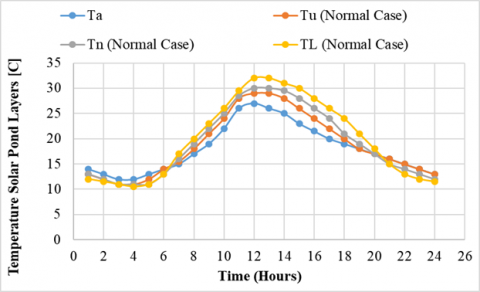Figure 2. Temperature of the three layers of the normal solar pond

## 3.pngFigure 3. The three-layer salinity solar pond's temperatures

## 4.png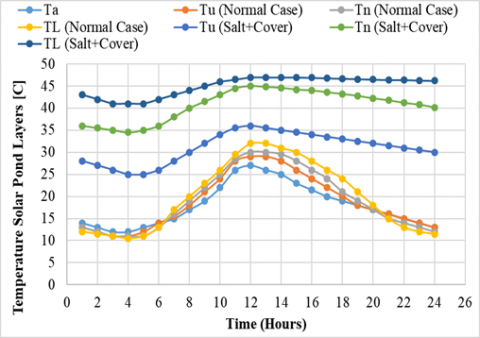Figure 4. Temperature of the three layers of the covered salty solar pond

Because the solar radiation amount on the surface of the pond affects the quantity of thermal energy kept in the layers of the pond, any increase in the radiation shed on the pond’s surface shows a growth in the thermal energy stored in the pond. This is where the reflective mirrors on the sides of the pond come into play, boosting the amount of radiation by redirecting the sun’s rays away from its surface and toward the surface of the pond. This is depicted in Figure 5.

## 5.png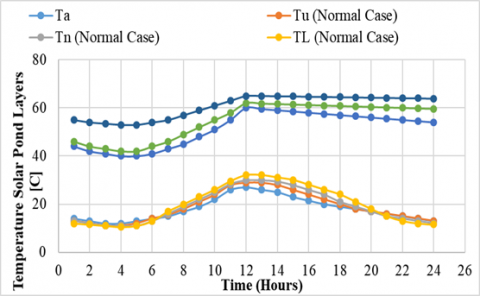Figure 5. Temperature of the three layers of the covered salty solar pond with the reflectors

Theoretical results using additive methods are shown in Figure 6 for usable energy in a solar pond with a normal pond and a salt gradient pond. Given that the reflective mirrors and cover raised the pool's temperature, more usable energy would almost certainly be generated. This is because it is directly related to temperature. According to the theoretical results, the pond has a usable power of 2 kW. Salts are added to this quantity to reach 7.5 kilowatts. The highest value of usable energy during the study is close to 20 kW due to the increase in radiation that reaches the pond surface caused by the addition of salts, reflecting mirrors and cover. The substrate's internal heat storage system benefits from the addition of salts as well.

## 6.png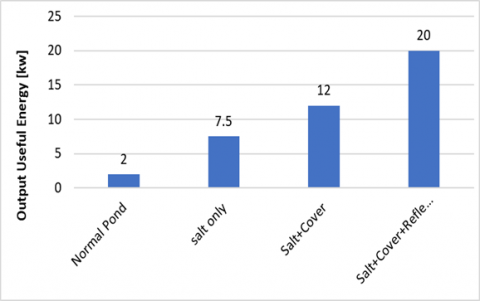Figure 6. Output useful energy of solar pond [kw]

Figure 7 displays the efficiency outcomes of the solar-powered pond with a salinity gradient and the standard pond. Adding gravel, cover, and reflective mirrors has made the pond more efficient. The efficacy of the ponds is increased by this technique because it improves the amount of energy that can be used by the solar pond, which is directly proportional to the solar pond’s thermal efficiency. The solar pond’s efficiency increased with improvements, going from 5% to 28.4%.

## 7.pngFigure 7. Output overall thermal efficiency of solar pond [%]

Figure 8 displays the current produced by TEG because of temperature differences and for three water flow rates via the tubes in contact with the electrodes. (TEG). It should be noted that the current value increases along with the flow rate. Because all the water in contact with the TEG at high speeds is of the same intensity, all the TEG chips function at the same productivity. Regarding the growth and its cause, Figure 9 shows behavior similar to that of the prior graph. The electrical potential differential (TEG) for three different water flow rates is depicted on the graph. According to the same principle, the increased flow rate happens as the electric potential difference widens. The electric power is calculated from the current and electrical potential difference induced by the flow of hot and cold water at three different flow rates on both sides of the TEG (TEG). This is depicted in Figure 10.

## 8.png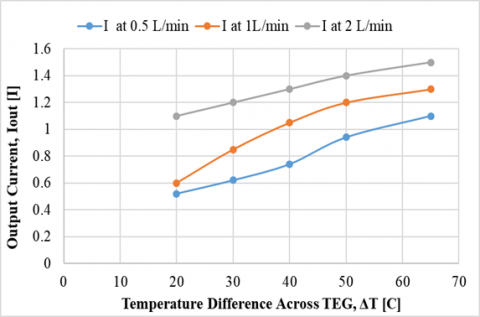Figure 8. The output current, Iout [A] for temperature difference across TEG, ΔT [C]

## 9.png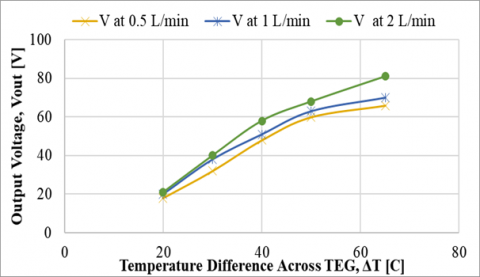Figure 9. The output voltage, Vout [V] for temperature difference across TEG, ΔT [C]

## 10.png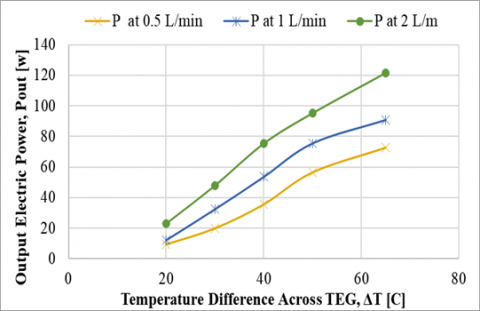Figure 10. The output electric power, Pout [w] for temperature difference across TEG, ΔT [C]

The electrical efficiency of the TEG is calculated using the electrical energy produced by the TEG and three flow rates, as shown in Figure 11. Since it can be seen in the figure that increasing mass flow rate enhances thermal efficiency, the best mass flow rate throughout the tests was 2 liters per minute.

## 11.png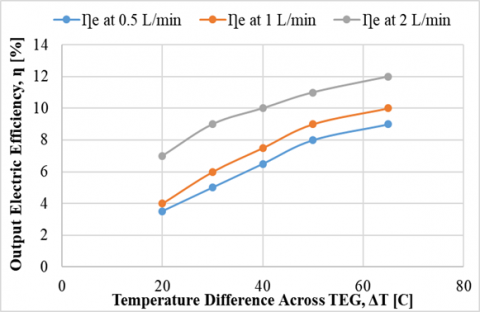Figure 11. The output electric efficiency, ƞ [%] for temperature difference across TEG, ΔT [C]

5. Conclusions

According to the theoretical findings got with the help of the Matlab software:

(1) While reflective mirrors help to raise the intensity of solar radiation directed at the pond’s surface, which serves to raise the amount of heat energy absorbed through the pond’s layers, the addition of salt helps to increase the amount of heat energy stored in the lower layer.

(2) The plastic cover that has been added to the pond’s surface helps to cut down on thermal energy losses.

(3) When salt, a cover, and mirrors were added, the quantity of useful energy increased from 2 kilowatts to 20 kilowatts when compared to the conventional pond.

(4) These improvements allowed the pond’s thermal efficiency to rise from 5 percent to 28.4 percent.

(5) TEG efficiency was 12% at a temperature of 65℃ and a mass flow rate of 2 L/min.

References

 Ali, M.M., Ahmed, O.K., Abbas, E.F. (2020). Performance of solar pond integrated with photovoltaic/thermal collectors. Energy Reports, 6: 3200-3211. https://doi.org/10.1016/j.egyr.2020.11.037

 Jassim, M.M., Abbood, M.H., Rashid, F.L. (2022). Design and construction solar oven sterilizer. International Journal of Heat and Technology, 40(2): 641-645.‏ https://doi.org/10.18280/ijht.400235

 Rasheed, R.M., Jabbar, S.A.A.S., AL Hussiny, N.M., Rasheed, A.M. (2021). Study of optimum performance of solar still process under weather conditions of Baghdad. International Journal of Nonlinear Analysis and Applications, 12(Special Issue): 1355-1366. https://doi.org/10.22075/ijnaa.2021.5712

 Al-Musawi, O.A., Khadom, A.A., Manhood, H.B., Mahdi, M.S. (2020). Solar pond as a low grade energy source for water desalination and power generation: A short review. Renewable Energy and Environmental Sustainability, 5: 4.‏ https://doi.org/10.1051/rees/2019008

 Panchal, H., Sadasivuni, K.K., Essa, F.A., Shanmugan, S., Sathyamurthy, R. (2021). Enhancement of the yield of solar still with the use of solar pond: A review. Heat Transfer, 50(2): 1392-1409. https://doi.org/10.1002/htj.21935

 Mamur, H., Dilmaç, Ö.F., Begum, J., Bhuiyan, M.R.A. (2021). Thermoelectric generators act as renewable energy sources. Cleaner Materials, 2: 100030.‏ https://doi.org/10.1016/j.clema.2021.100030

 Ding, L.C., Akbarzadeh, A., Tan, L. (2018). A review of power generation with thermoelectric system and its alternative with solar ponds. Renewable and Sustainable Energy Reviews, 81: 799-812. https://doi.org/10.1016/j.rser.2017.08.010

 Hakim, I.I., Putra, N., Ananda, Y.O. (2020). Investigation on the use solar thermoelectric generator for open pond cultivation with heat pipe cooling. Engineering Journal, 24(4): 295-304. https://doi.org/10.4186/ej.2020.24.4.295

 Wicaksono, R.M., Yunesti, P. (2021). Study of thermoelectric generator utilization to recover heat at low temperature grade application: A review. Journal of Science and Applicative Technology, 5(2): 313-318.

 Özbaş, E. (2019). Experimental investigation of passive water cooling in solar heating thermoelectric generator. Politeknik Dergisi, 23(4): 1231-1236. https://doi.org/10.2339/politeknik.613095

 Khalilian, M. (2017). Experimental investigation and theoretical modelling of heat transfer in circular solar ponds by lumped capacitance model. Applied Thermal Engineering, 121: 737-749. https://doi.org/10.1016/j.applthermaleng.2017.04.129

 Assari, M.R., Tabrizi, H.B., Nejad, A.K., Parvar, M. (2015). Experimental investigation of heat absorption of different solar pond shapes covered with glazing plastic. Solar Energy, 122: 569-578. https://doi.org/10.1016/j.solener.2015.09.013

 Mahdi, J.T., Jebbar, Y.A. (2019). A theoretical investigation of solar radiation and heat transfer in a solar pond in Karbala city. In AIP Conference Proceedings, 2144(1): 030019. https://doi.org/10.1063/1.5123089

 Sogukpinar, H. (2019). Seasonal temperature variation of solar pond under Mediterranean condition. Thermal Science, 23(6 Part A): 3317-3326. ‏ https://doi.org/10.2298/TSCI180518060S

 Rizvi, R.Z., Jamal, Y., Ghauri, M.B., Salman, R., Khan, I. (2015). Solar pond technology for brine management and heat extraction: A critical review. Journal of Faculty of Engineering & Technology, 22(2): 69-79. ‏

 Kumar, A., Singh, K., Verma, S., Das, R. (2018). Inverse prediction and optimization analysis of a solar pond powering a thermoelectric generator. Solar Energy, 169: 658-672. https://doi.org/10.1016/j.solener.2018.05.035

 Verma, S., Das, R. (2020). Revisiting gradient layer heat extraction in solar ponds through a realistic approach. Journal of Solar Energy Engineering, 142(4): 041009. https://doi.org/10.1115/1.4046149

 Abbood, M.H., Alhwayzee, M., Sultan, M.A. (2021). Experimental investigation into the performance of the solar pond in Kerbala. In IOP Conference Series: Materials Science and Engineering, 1067(1): 012098. https://doi.org/10.1088/1757-899X/1067/1/012098

 Jouhara, H., Żabnieńska-Góra, A., Khordehgah, N., Doraghi, Q., Ahmad, L., Norman, L., Dai, S. (2021). Thermoelectric generator (TEG) technologies and applications. International Journal of Thermofluids, 9: 100063. ‏https://doi.org/10.1016/j.ijft.2021.100063

 Allouhi, A. (2019). Advances on solar thermal cogeneration processes based on thermoelectric devices: A review. Solar Energy Materials and Solar Cells, 200: 109954. https://doi.org/10.1016/j.solmat.2019.109954

 Hashim, H.T., Rashid, F.L., Kadham, M.J. (2021). Concentration solar thermoelectric generator (CSTEG): Review paper. Journal of Mechanical Engineering Research and Developments, 44(1): 435-447.

 Hassan Abbood, M., Rashid, F.L., Jassim, M.M. (2022). Design and construction solar steam sterilizer. International Journal of Nonlinear Analysis and Applications, 13(1): 2535-2547. https://doi.org/10.22075/ijnaa.2022.5955

 Rashid, F.L., Fayyadh, I.K., Hashim, A. (2012). Design of solar pond for electricity production. British Journal of Science, 3(2).